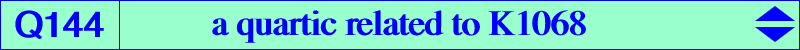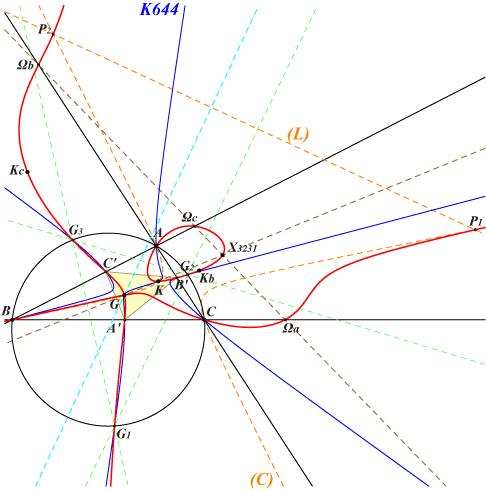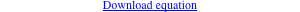too complicated to be written here. Click on the link to download a text file.X(2), X(6), X(3231), X(5000), X(5001) vertices of the cevian triangle A'B'C' of X(83) vertices of the tangential triangle KaKbKc vertices of the Grebe triangle G1G2G3 centers Ωa, Ωb, Ωc of the Apollonius circles other points belowK1068 is the transform of the trilinear polar (L) of X(83) under the mapping 𝚹 : P -> aQ described in page K1065 where further informations and a generalization are given. The transform of Q144 is also K1068. Q144 is a nodal quartic analogous to Q098 which is obtained when the line (L) is the line at infinity, both transformed under 𝚹 into K028. *** Q144 meets the A-symmedian of ABC at A, K, Ka and a fourth point A1 which also lies on the line GΩa, B1 and C1 being defined likewise. Q144 meets (L) at two imaginary points on the circumcircle and two (always real) points P1, P2 on the circum-conic (C) with perspector X(512) passing through G and K. The circle with diameter P1P2 is orthogonal to (O) and its center is S = (-2 a^2 + b^2 + c^2)(b^2 - c^2) / (b^2 + c^2) : : , SEARCH = -9.83721810368216, also on the lines {99,827}, {115,804}, etc. S is now X(22105) in ETC (2018-09-04). Note that Q144 and each one of the following cubics share 12 identified points : • K644 = pK(X251, X83) shares A, B, C, A', B', C', G1, G2, G3, K and G (twice). • K102 = pK(X6, X6) shares A, B, C, G (each counted twice), G1, G2, G3 and K. • K177 = pK(X32, X2) shares A, B, C, Ka, Kb, Kc, G1, G2, G3, K and G (twice).